International Tables for Crystallography (2006). Vol. F, ch. 26.1, pp. 745-772   | 1 | 2 | https://doi.org/10.1107/97809553602060000725

Contents

• 26.1. How the structure of lysozyme was actually determined  (pp. 745-772)
• 26.1.1. Introduction  (p. 745) | html | pdf |
• 26.1.2. Structure analysis at 6 Å resolution  (pp. 745-753) | html | pdf |
• 26.1.2.1. Technical facilities  (p. 745) | html | pdf |
• 26.1.2.2. Lysozyme crystallization  (pp. 745-746) | html | pdf |
• 26.1.2.3. Preparation of heavy-atom derivatives  (p. 746) | html | pdf |
• 26.1.2.4. Determination of heavy-atom positions  (pp. 746-747) | html | pdf |
• 26.1.2.4.1. The mercuri-iodide (K 2 HgI 4 ) derivative  (p. 747) | html | pdf |
• 26.1.2.4.2. The palladium chloride (K 2 PdCl 4 ) derivative  (p. 747) | html | pdf |
• 26.1.2.4.3. The o -mercurihydroxytoluene p -sulfonate (MHTS) derivative  (p. 747) | html | pdf |
• 26.1.2.4.4. Other potential derivatives  (p. 747) | html | pdf |
• 26.1.2.5. Refinement of heavy-atom parameters  (p. 747) | html | pdf |
• 26.1.2.6. Analysis in three dimensions  (pp. 747-751) | html | pdf |
• 26.1.2.6.1. X-ray intensity measurements  (pp. 747-750) | html | pdf |
• 26.1.2.6.2. Data processing  (pp. 750-751) | html | pdf |
• 26.1.2.6.3. The absolute scale of the intensities  (p. 751) | html | pdf |
• 26.1.2.6.4. Re-assessment of heavy-atom derivatives  (p. 751) | html | pdf |
• 26.1.2.7. Phase determination at 6 Å resolution  (pp. 751-752) | html | pdf |
• 26.1.2.8. The electron-density map of lysozyme at 6 Å resolution  (pp. 752-753) | html | pdf |
• 26.1.3. Analysis of the structure at 2 Å resolution  (pp. 753-765) | html | pdf |
• 26.1.3.1. Heavy-atom derivatives at 2 Å resolution  (pp. 754-756) | html | pdf |
• 26.1.3.2. Intensity measurements  (pp. 756-757) | html | pdf |
• 26.1.3.3. The second low-resolution map at 6 Å  (p. 757) | html | pdf |
• 26.1.3.4. Intensity measurements at high resolution  (pp. 757-759) | html | pdf |
• 26.1.3.4.1. Experimental methods  (pp. 757-758) | html | pdf |
• 26.1.3.4.2. Diffractometer output  (pp. 758-759) | html | pdf |
• 26.1.3.5. Data processing  (p. 759) | html | pdf |
• 26.1.3.5.1. Absorption corrections  (p. 759) | html | pdf |
• 26.1.3.6. Further stages of data processing  (pp. 759-760) | html | pdf |
• 26.1.3.7. The crystal-type problem  (pp. 760-761) | html | pdf |
• 26.1.3.8. Final refinement of heavy-atom parameters  (pp. 761-762) | html | pdf |
• 26.1.3.9. Calculation of phase values  (p. 762) | html | pdf |
• 26.1.3.10. The electron-density map at 2 Å resolution  (p. 763) | html | pdf |
• 26.1.3.11. Map interpretation and model building  (pp. 763-765) | html | pdf |
• 26.1.4. Structural studies on the biological function of lysozyme  (pp. 765-769) | html | pdf |
• 26.1.4.1. Lysozyme substrates  (pp. 765-766) | html | pdf |
• 26.1.4.2. The crystal structure of GlcNAc  (p. 766) | html | pdf |
• 26.1.4.3. Low-resolution binding studies of lysozyme with GlcNAc and other sugars  (p. 766) | html | pdf |
• 26.1.4.4. Binding studies of lysozyme with tri- N -acetyl-chitotriose, (GlcNAc) 3 , at 2 Å resolution  (pp. 766-768) | html | pdf |
• 26.1.4.5. Proposals for the catalytic mechanism of lysozyme  (pp. 768-769) | html | pdf |
• References | html | pdf |
• Figures
• Fig. 26.1.2.1. Tetragonal lysozyme crystals with well developed {110} faces (left-hand crystal) and small {110} faces (right-hand crystal)  (p. 746) | html | pdf |
• Fig. 26.1.2.2. Difference-Patterson h 0 l projection map for the derivative obtained with K 2 PdCl 4   (p. 747) | html | pdf |
• Fig. 26.1.2.3. Difference-Patterson hk 0 projection for the derivative obtained with MHTS  (p. 747) | html | pdf |
• Fig. 26.1.2.4. Reciprocal-space diagrams showing the direction of the incident X-ray beam, the Ewald sphere and the genesis of a reflection ( a ) in an equatorial plane and ( b ) in the equi-inclination setting  (p. 748) | html | pdf |
• Fig. 26.1.2.5. Crystal mounting  (p. 749) | html | pdf |
• Fig. 26.1.2.6. Absorption curve  (p. 749) | html | pdf |
• Fig. 26.1.2.7. Typical output from the linear diffractometer  (p. 750) | html | pdf |
• Fig. 26.1.2.8. Format of monitor output in which the computer lists reflections that fail the tests for format or significance  (p. 750) | html | pdf |
• Fig. 26.1.2.9. Three-dimensionalsyntheses for the PdCl 4 and MHTS derivatives  (p. 751) | html | pdf |
• Fig. 26.1.2.10. Phase determination for reflection 424  (p. 752) | html | pdf |
• Fig. 26.1.2.11. Electron-density difference syntheses showing the main sites of substitution and subsidiary sites with low occupancy in the PdCl 4 and MHTS derivatives (Fenn, 1964)  (p. 753) | html | pdf |
• Fig. 26.1.2.12. Electron-density distribution in lysozyme at 6 Å resolution viewed parallel to the c axis  (p. 753) | html | pdf |
• Fig. 26.1.2.13. Views of a 6 Å resolution model of the regions in which the electron density exceeds about 0.53 e Å −3   (p. 754) | html | pdf |
• Fig. 26.1.3.1. Perspective drawing of the sphere of reflection showing inclination geometry with simultaneous reflections (reciprocal-lattice points P and Q) from levels symmetrically related by the flat-cone setting  (p. 756) | html | pdf |
• Fig. 26.1.3.2. Theplane of the reciprocal lattice oriented to rotate about theaxis  (p. 757) | html | pdf |
• Fig. 26.1.3.3. Solid model of the electron density greater than about 0.5 e Å −3 in the second study of lysozyme at 6 Å resolution  (p. 758) | html | pdf |
• Fig. 26.1.3.4. Paper-tape output from the triple-counter linear diffractometer, showing the indices of the central reflection and the background, peak, background counts for the three reflections  (p. 759) | html | pdf |
• Fig. 26.1.3.5. Asymmetric mounting of a protein crystal with its mother liquor in a capillary tube  (p. 760) | html | pdf |
• Fig. 26.1.3.6. Plot of the ratio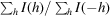against h   (p. 760) | html | pdf |
• Fig. 26.1.3.7. ( a ) Phase probability curve for a Bijvoet pair of reflections (broken lines) with the joint probability curve (full line) derived by the method of Blow & Rossmann (1961)  (p. 762) | html | pdf |
• Fig. 26.1.3.8. Variation of the mean figure of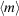with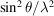(p. 763) | html | pdf |
• Fig. 26.1.3.9. Photograph of sections z = 35/60 to 44/60 of the three-dimensional electron-density map of hen egg-white lysozyme at 2 Å resolution  (p. 763) | html | pdf |
• Fig. 26.1.3.10. The amino-acid sequence of hen egg-white lysozyme (Canfield & Liu, 1965)  (p. 764) | html | pdf |
• Fig. 26.1.3.11. Schematic drawing of the main-chain conformation of lysozyme  (p. 764) | html | pdf |
• Fig. 26.1.3.12. Stereo-photographs of a model of the lysozyme molecule to a scale of 2 cm to 1 Å  (p. 765) | html | pdf |
• Fig. 26.1.4.1. The cell-wall tetrasaccharide with the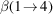glycosidic bond that is hydrolysed by lysozyme indicated (Blake et al   (p. 765) | html | pdf |
• Fig. 26.1.4.2. Inhibitor molecules of lysozyme (Blake et al   (p. 767) | html | pdf |
• Fig. 26.1.4.3. Contemporary drawings of the binding to lysozyme of: ( a ) β- N -acetylglucosamine and ( b ) α- N -acetylglucosamine (Blake et al   (p. 768) | html | pdf |
• Fig. 26.1.4.4. Stereo-photographs of a model of the lysozyme molecule showing how a hexasaccharide substrate may bind to the enzyme  (p. 769) | html | pdf |
• Fig. 26.1.4.5. Draft sketch of the lysozyme–hexasaccharide substrate complex prepared by Irving Geis and annotated by David Phillips for an article published in Scientific American in 1966 (Phillips, 1966)  (p. 770) | html | pdf |
• Tables
• Table 26.1.2.1. Heavy-atom parameters used in the final phase calculation for the lysozyme structure  (p. 752) | html | pdf |
• Table 26.1.3.1. Structure amplitudes of the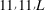reflections from crystal types I and II  (p. 761) | html | pdf |
• Table 26.1.3.2. Heavy-atom parameters for the 2 Å structure  (p. 761) | html | pdf |
• Table 26.1.3.3. Discrepancies in amino-acid sequences (excluding Asp/Asn)   (p. 764) | html | pdf |